کمیت: 0

مجموع: 0,00

0

# Surface area of spheres (demonstration)

### Surface area of spheres (demonstration)

The surface of a sphere consists of the set of points which are all at the same distance from a given point in space.

رياضى

کلیدواژه‌ها

surface of sphere, great circle, mathematics, geometry, surface

موارد مربوط

### نماها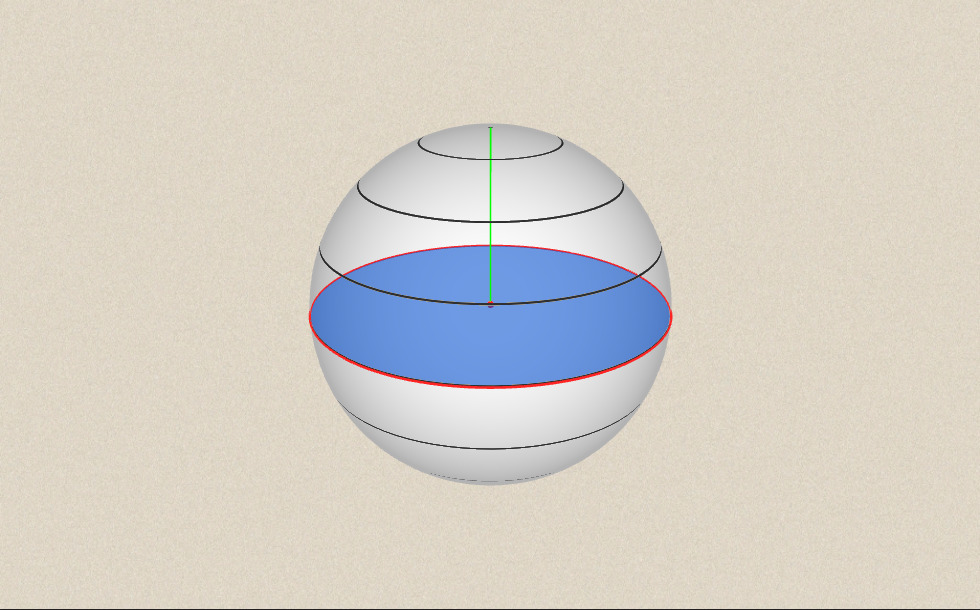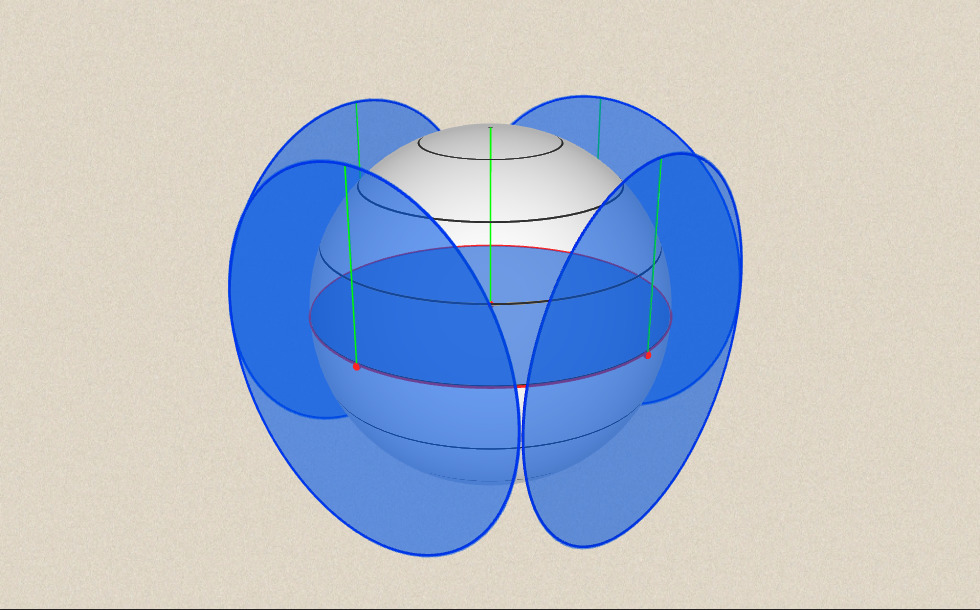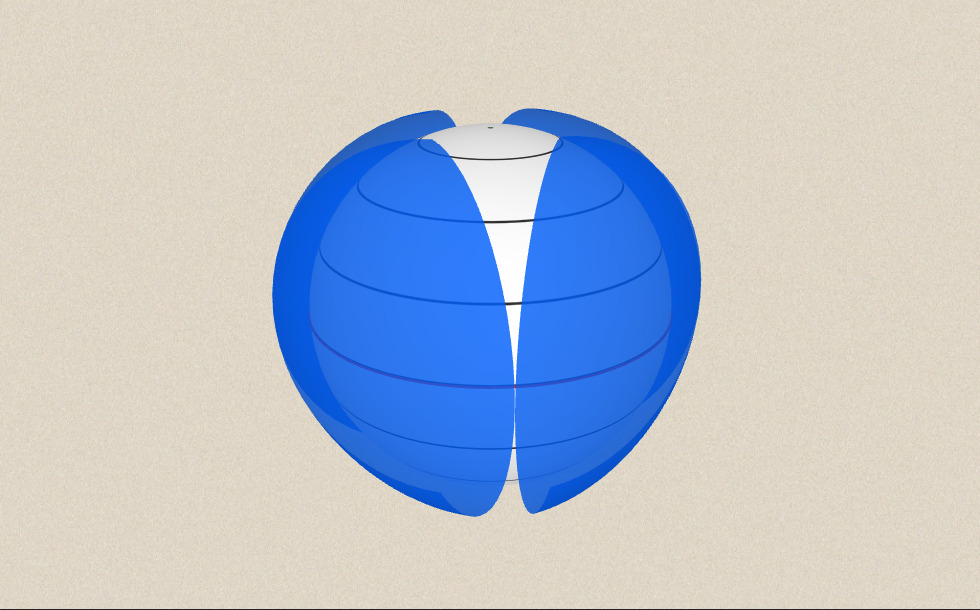### موارد مربوط#### Sphere

A sphere is the set of points which are all within the same distance from a given point in space.#### محیط، مساحت، سطح و حجم

این انیمیشن فرمول های محاسبه محیط و مساحت شکل ها و همچنین مساحت و حجم احجام هندسی را نمایش می دهد۔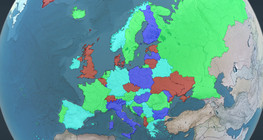#### Four colour theorem

Colour a map with the fewest number of colours possible, so that no two adjacent regions have the same colour.#### Non-orientable surfaces

The Möbius strip and the Klein bottle are special two-dimensional surfaces with only one side.#### Volume of spheres (Cavalieri´s principle)

Calculating the volume of a sphere is possible using an appropriate cylinder and cone.#### Volume of spheres (demonstration)

The sum of the volume of the ´tetrahedrons´ gives an approximation of the volume of the sphere.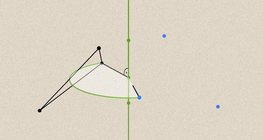#### تغییرات هندسی - چرخش

این انیمیشن چرخش هندسی را در صفحه و فضا نشان می دهد۔#### Solids of revolution

Rotating a geometric shape around a line within its geometric plane as an axis results in a solid of revolution.